Theis Equation Calculator

Compute drawdown for unsteady groundwater flow to a pumping well in a confined aquifer

Register to enable "Calculate" button in Theis drawdown calculator.

 www.LMNOeng.com Pumping rate, Q: m3/s m3/min m3/hr m3/day ft3/s ft3/min ft3/hr ft3/day gal/min (US) gal/hr (US) gal/day (US) Radial distance from pumped well, r: cm m km inch feet miles Time since pumping began, t: seconds minutes hours days years Aquifer transmissivity, T: m2/s m2/min m2/hr m2/day ft2/s ft2/min ft2/hr ft2/day gpm/ft (US) gph/ft (US) gpd/ft (US) Storage coefficient, Sc: u = r2 Sc/(4 T t): Well function, W: Drawdown, s: cm m inch ft © 2017 LMNO Engineering, Research, and Software, Ltd.

Units in Theis unsteady groundwater flow to a well calculator: cm=centimeter, ft=foot, gal=gallon (U.S.), hr=hour, km=kilometer, m=meter, min=minute, s=second.

Diagrams of Groundwater Flow to a Pumping Well (Wells Screened in Confined Aquifer)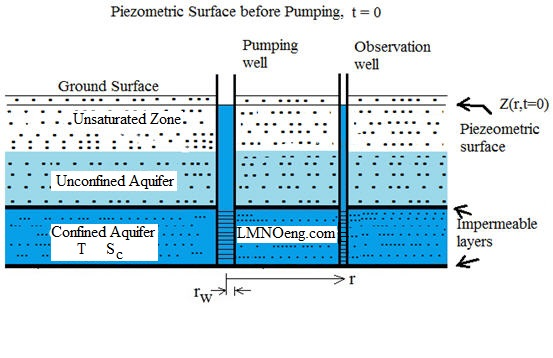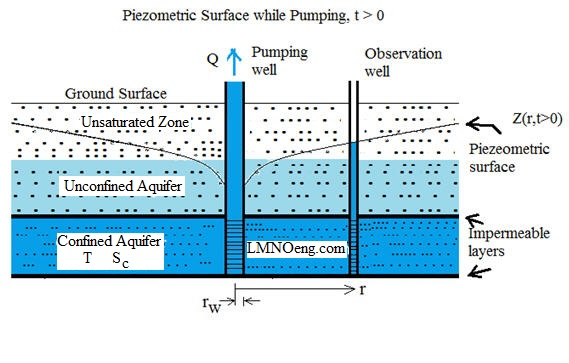Unsteady Groundwater Flow to a Pumping Well in a Confined Aquifer
Pumping water out of (or into) a confined aquifer is a common occurrence. Our calculation computes the change in the piezometric surface as water is pumped. In a confined aquifer, the piezometric surface is the elevation of the water surface in an observation well drilled into the confined aquifer and screened in the confined aquifer. The water level rises above the upper confining layer.

Drawdown s is the difference between the elevation of the piezometric surface prior to pumping Z(r,t=0) and the elevation of the piezometric surface during pumping Z(r,t>0). Thus:

s(r,t) = Z(r,t=0) - Z(r,t>0).

Equation - Conservation of Mass
Unsteady groundwater flow to a pumping well in a confined aquifer is described by the equation (Bear, 1979, p. 320):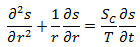The equation describes mass conservation of groundwater flow in the radial direction allowing for change in storage volume (expansion or contraction of the confined water due to change in pressure).

Boundary Conditions
Pumping rate Q is positive but has a negative sign in the boundary condition equation below since water goes toward the well when it is pumped. Water flowing toward the well is flowing in the negative r direction. Thus, to define Q as positive when pumping water out of the aquifer, the boundary condition needs a negative sign:

s(r=0,t=0) = 0     s(r=∞,t) = 0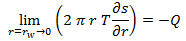The goal is to solve for drawdown as a function of radial distance r from the pumping well at time t since pumping started. The solution is commonly called the Theis solution, after Charles Theis who developed the solution in 1935 (Bear, 1979, p. 321):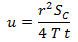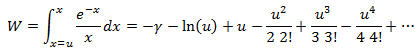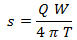Our calculation carries out the series to the u100 term which is sufficient for convergence to machine precision for u up to 9.5.

The LMNO Engineering unsteady groundwater flow calculation allows entry of either a positive or negative value for the pumping rate Q. A positive Q simulates pumping, and drawdown s will be positive since the piezometric surface drops. If a negative value is entered for Q, water injection is simulated. In that case drawdown is negative since the piezometric surface rises.

The groundwater flow program checks that r, t, T, and Sc are positive inputs. It also checks to make sure u ≤ 9.5 which is the maximum value printed in many texts (e.g. Bear, 1979, pp. 320-321). All input variables are converted to SI units prior to calculations.

The groundwater calculation does not check if the input values are physically reasonable and does not check if Reynolds number Re is less than or equal to 1.0. Darcy's law for groundwater flow (expressed in the boundary condition above that has "-Q" in it) is only valid for Re ≤ 1. Darcy's law is typically valid in groundwater flow since water velocities are very low through porous subsurface formations.

Notation
Units shown in notation are SI. The groundwater calculation converts user-selected units to SI, performs calculations in SI, then converts output variables to user-selected units.
Q = Steady pumping rate (m3/s).
r = Radial distance from centerline of pumping well (m).
rw = Pumping well radius (m).
s = Drawdown (m).
Sc = Confined aquifer storage coefficient.
t = Time since beginning of pumping (s).
T = Confined aquifer transmissivity (m2/s).
u = Collection of terms. Computed after all variables converted to SI units.
W = Theis well function.
Z = Elevation of piezometric surface (elevation that water rises to in observation well screened in confined aquifer) (m).
γ = Euler's constant = 0.5772156649... (Abramowitz & Stegun, 1972, p. 229).
π = Constant, 3.14159265...
ln( ) = Natural logarithm.
! = Factorial function.

References
Abramowitz, M. and Stegun, I. A. 1972. Handbook of Mathematical Functions with Formulas Graphs, and Mathematical Tables. Dover Publications, Inc.

Bear, J. 1979. Hydraulics of Groundwater. McGraw-Hill Publishing Co.

© 2017 LMNO Engineering, Research, and Software, Ltd.  All rights reserved.

Please contact us for consulting or other questions about groundwater flow.

LMNO Engineering, Research, and Software, Ltd.
7860 Angel Ridge Rd.   Athens, Ohio  45701  USA   Phone: (740) 592-1890
LMNO@LMNOeng.com    http://www.LMNOeng.com

To:
LMNO Engineering home page (more calculations)

Related Groundwater Calculations:

Permeameter

Compute gradient from well head measurements

1-D Step injection with advection, dispersion, retardation

3-D Pulse (slug) injection with advection, dispersion

Transmissivity T=Kb (and K table)

Register